# Stock Liao information

— Basic knowledge of stocks|Introduction to basics of stocks|Stock learning|Basic knowledge of stocks
Mobile access：m.liaochihuo.com

# Comic: Finding the Best Times to Buy and Sell Stocks

Release Time:2022-06-05 Topic:cartoon picture about rising stocks Reading：64 Navigation：Stock Liao information > educate > Comic: Finding the Best Times to Buy and Sell Stocks————— next day —————What does it mean? Let's take an example, given the following array:The stock rise and fall curve corresponding to this array is as follows: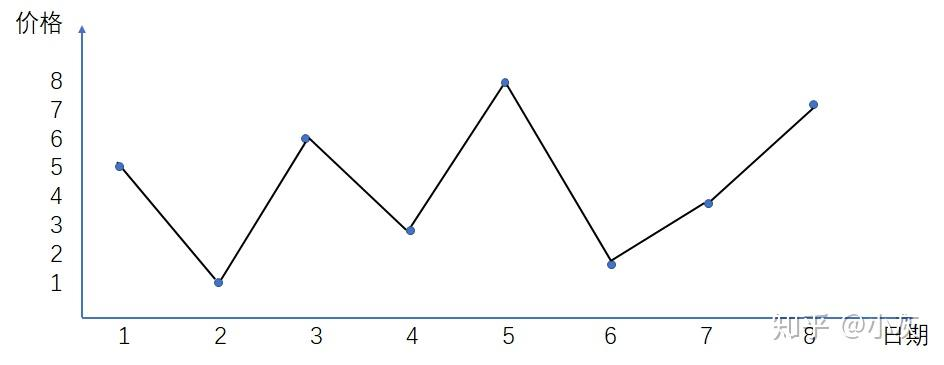p>

Obviously, buying from the second day when the price is 1 and selling from the fifth day when the price is 8 can get the maximum profit: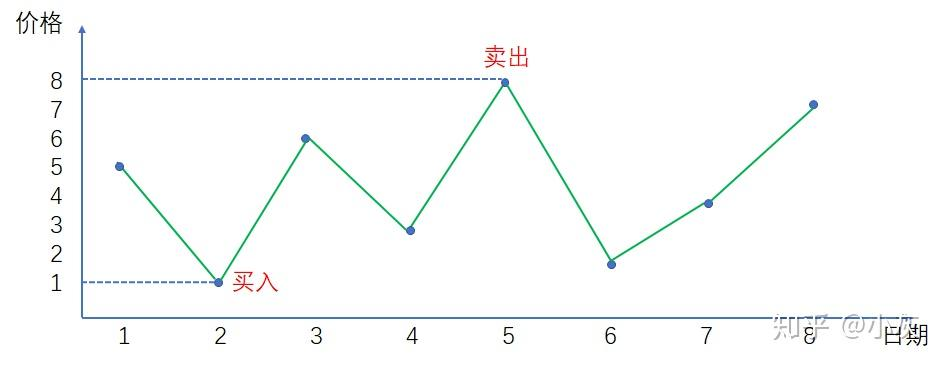The maximum gain at this time is 8-1=7 .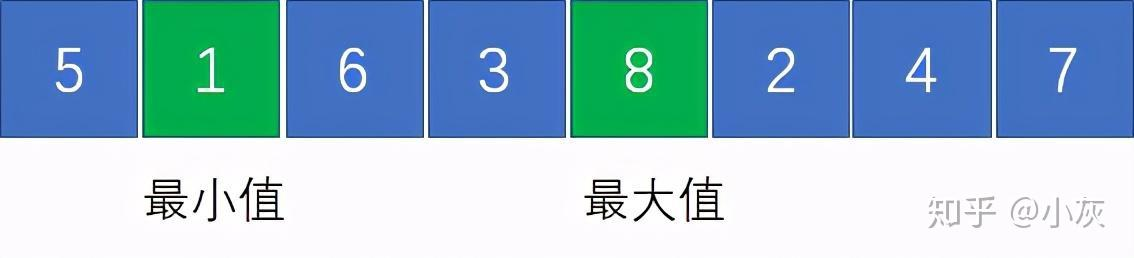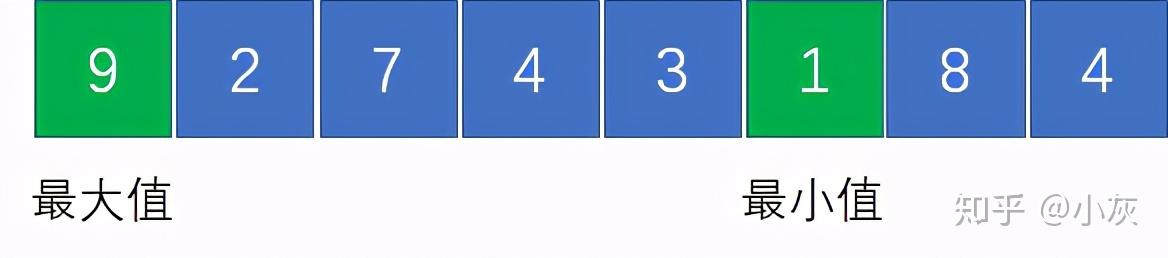In the above example, the maximum value of 9 is before the minimum value of 1, how should we trade? You can't turn back time, can you?————————————

p>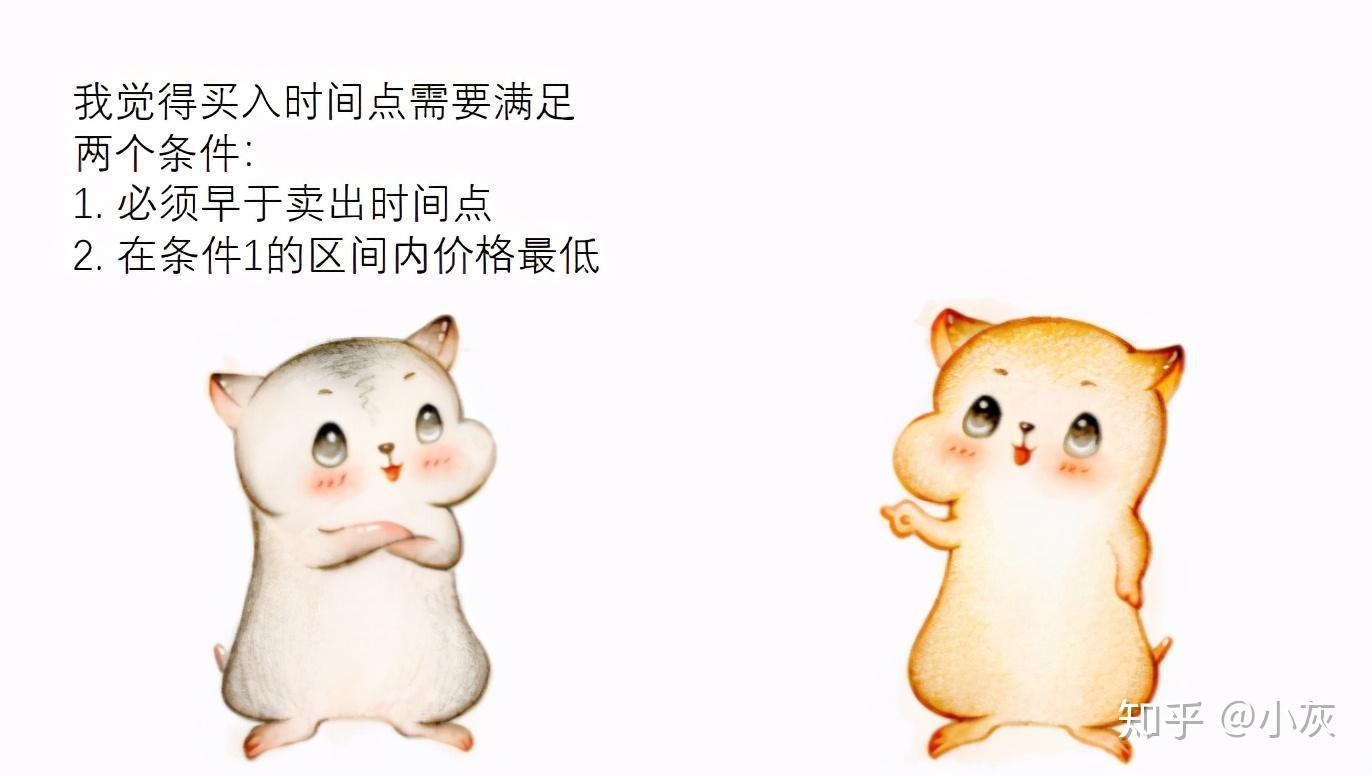The following picture is an example, if we have determined the price 4 is the time to sell, so what is the best time to buy at this time?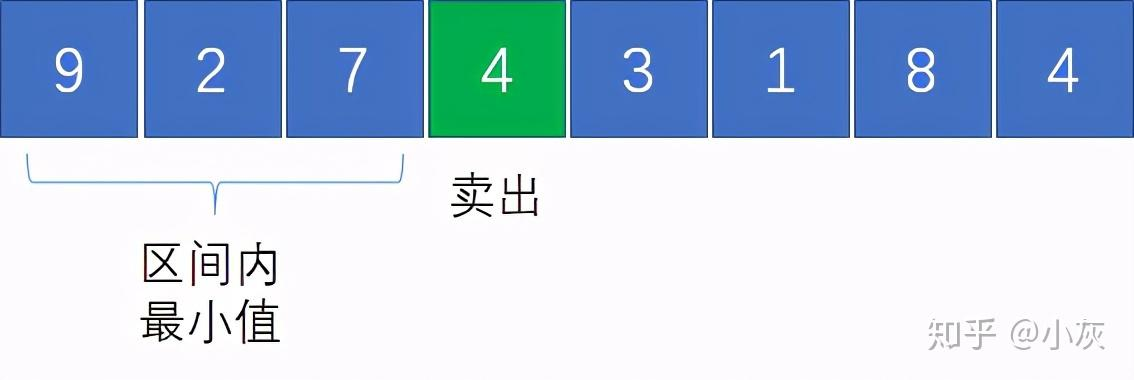We want to choose the interval before price 4, and it must be the minimum value in the interval. Obviously, the best choice is the time point of price 2.Step 1, initialize the operation, take the first element of the array as the temporary minimum price; the initial value of the maximum profit is 0: p>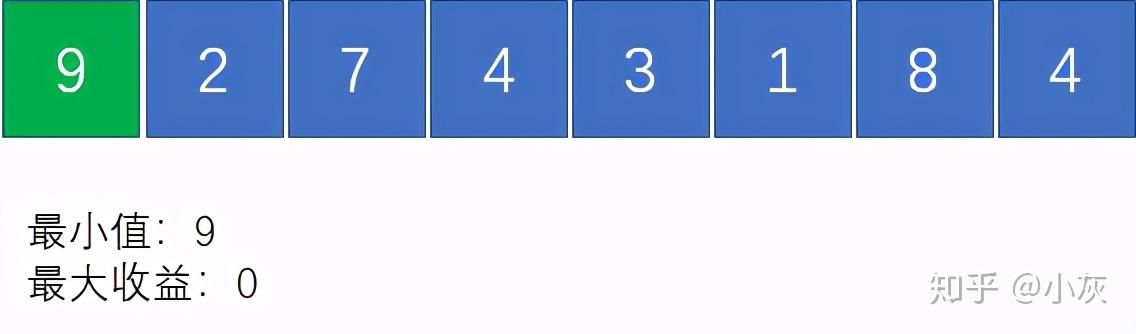Step 2 , traverse to the second element, due to 2

Step 3, traverse to the third element element, since 7>2, the current minimum price is still 2; as we just said, assuming price 7 is the selling point, the corresponding best buying point is price 2, and the difference between the two is 7-2=5 , 5>0, so the current maximum gain becomes 5: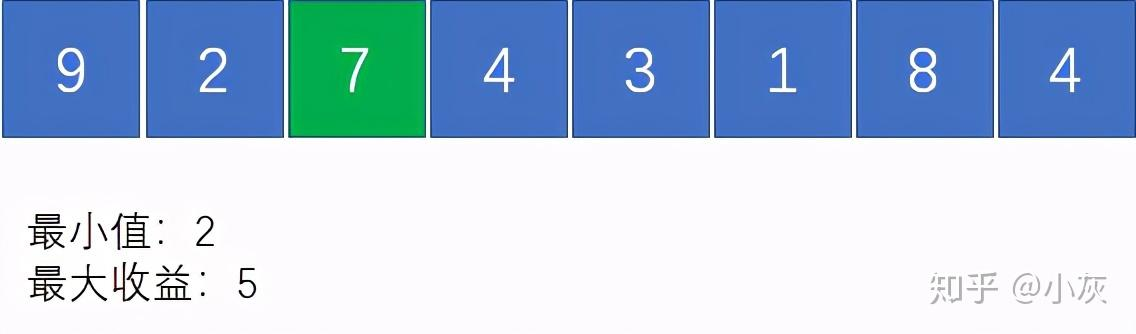Step 4, traverse to the 4th element, since 4>2, the current minimum price is still 2; 4-2=2, 2

Step 5, traverse to the 5th element, since 3>2, the current minimum price is still is 2; 3-2=1, 1

and so on, we traverse to the end of the array, the minimum price at this time is 1 ; maximum payoff is 8-1=7: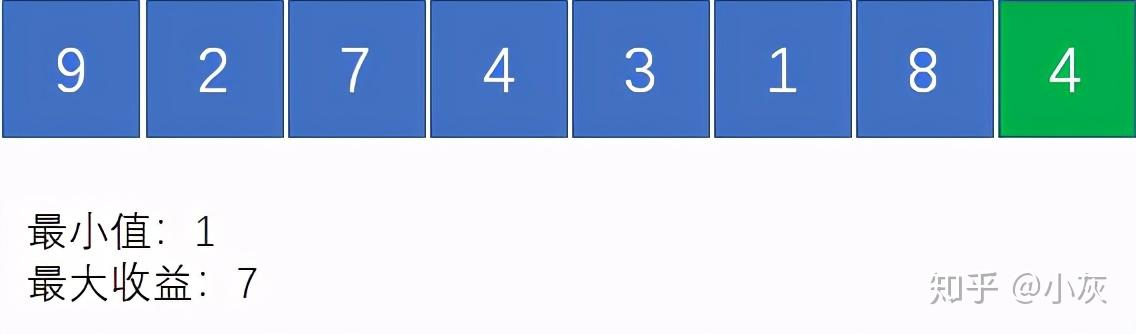public class StockProfit { public static int maxProfitFor1Time(int prices[ ]) { if(prices==null || prices.length==0) { return 0; } int minPrice = prices[ 0 ]; int maxProfit = 0; for (int i = 1; i < prices.length; i++) { if (prices[ i ] < minPrice) { minPrice = prices[ i ]; } else if(prices[ i ] - minPrice > maxProfit){ maxProfit = prices [ i ] - minPrice; } } return maxProfit; } public static void main(String[ ] args) { int[ ] prices = {9,2,7,4,3,1,8,4}; System.out. println(maxProfitFor1Time(prices)); }}Let's take the following array as an example to visually look at the buying and sellingTiming: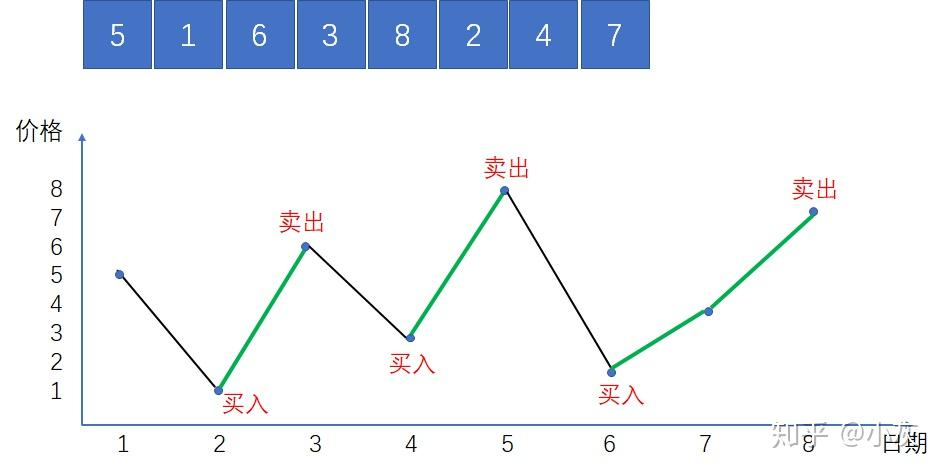in the picture , the green line segment represents the stage of price increase. Since there is no limit to the number of buys and sells, we can buy at every low and sell at every high.

In this way, all the green parts are our benefits, and the maximum total benefit is the sum of these partial benefits Total:

(6-1)+(8-3)+(4-2)+(7-4)= 15

As for how to identify these green rising stages? Very simple, we traverse the entire array, if the latter element is greater than the previous element, it means that the stock price is in the rising stage.public int maxProfitForAnyTime(int[ ] prices) { int maxProfit = 0; for (int i = 1; i < prices.length; i++) { if (prices[ i ] > prices[ i-1 ]) maxProfit += prices[ i ] - prices[ i-1 ]; } return maxProfit; }We still use the previous array as an example:First, find the best buying and selling point under the limit of 1 buy and sell: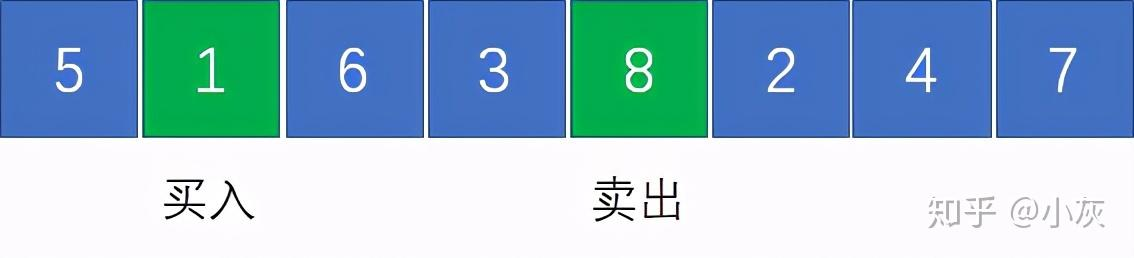The positions of the two trades cannot cross, so we find the first trade After the position, remove the pair of buy and sell points and the elements in between them: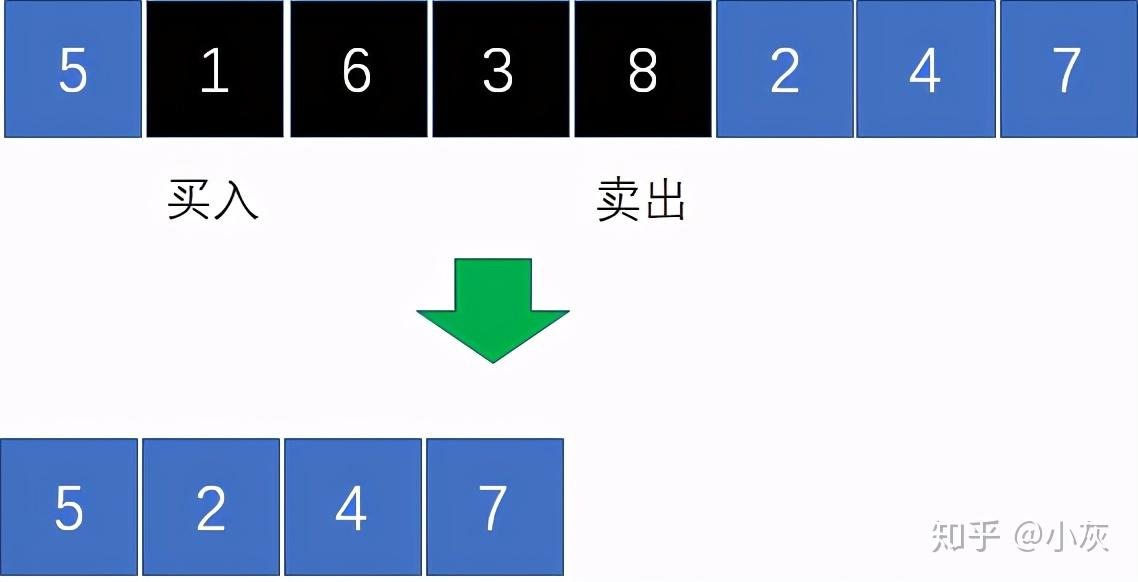Next, we follow the same idea and find the best buy and sell point for the second trade from the remaining elements: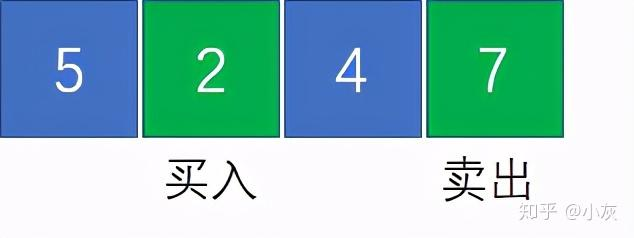This way, we found the most Best choice in case of 2 buys and sells: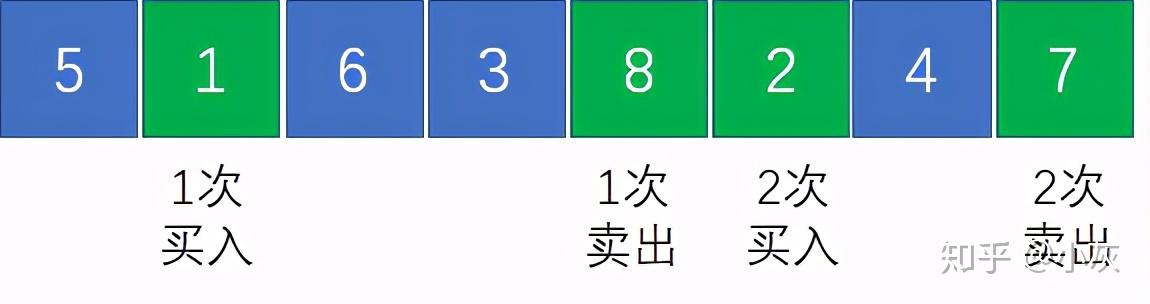For the array in the above figure, if it is solved twice independently, the best buy and sell The points are [1,9] and [6,7] respectively, and the maximum profit is (9-1)+(7-6)=9: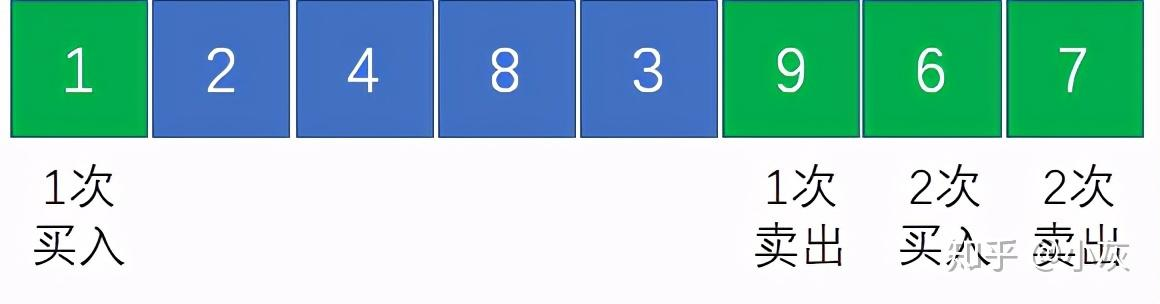But in fact, if you choose [1,8] and [3,9], the maximum profit is (8-1)+(9-3)=13>9: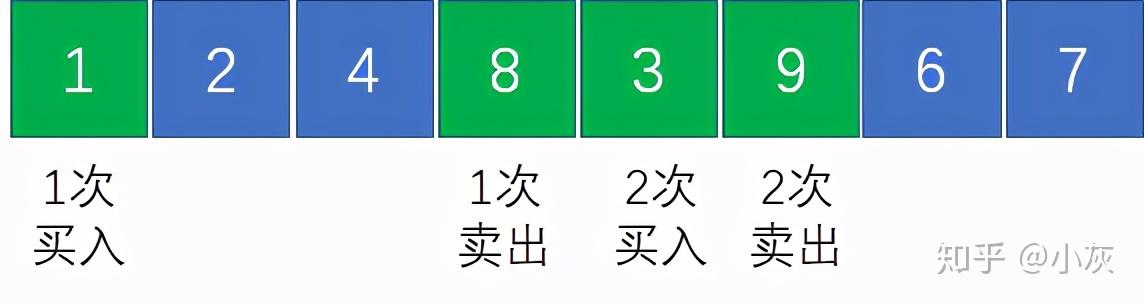When the first best buy and sell point is determined, the second buy and sell What are the situations of the exit point?

Case 1: The second best buy and sell Out point, to the left of the 1st buy and sell point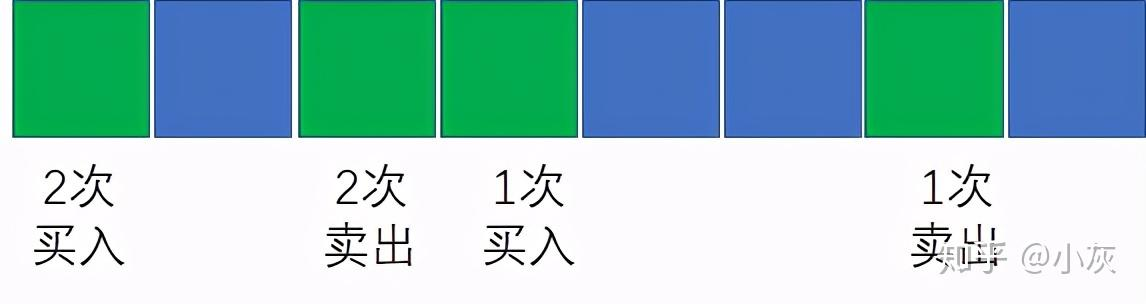< p data-pid="T73qV6AT">Case 2: The second best buy and sell point is to the right of the first buy and sell point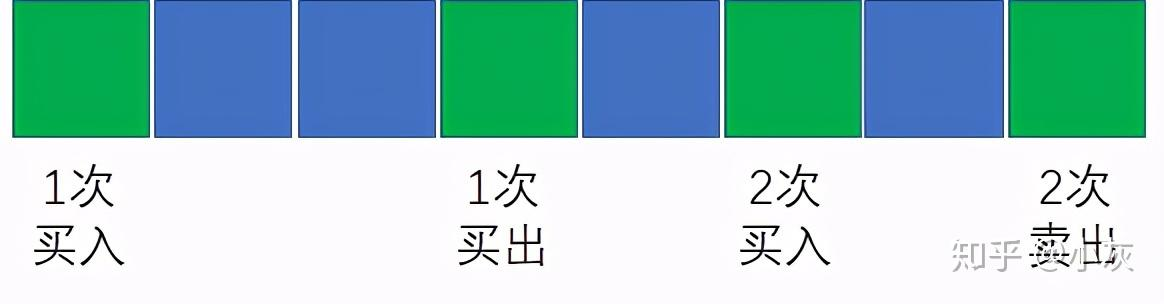Case 3: The first buying and selling interval is truncated from the middle and re-split into two Buy & Sell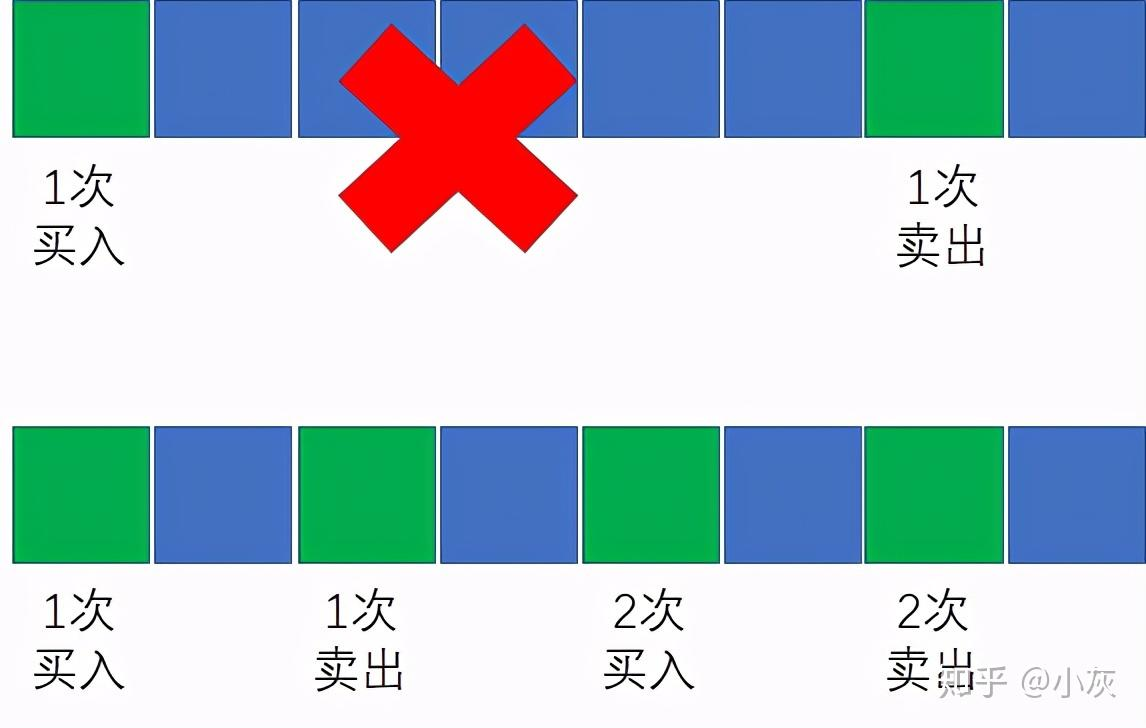So, How to judge which situation a given stock price array belongs to? It is very simple. After the first maximum buying and selling point is determined, we can compare which situation brings the greatest increase in income: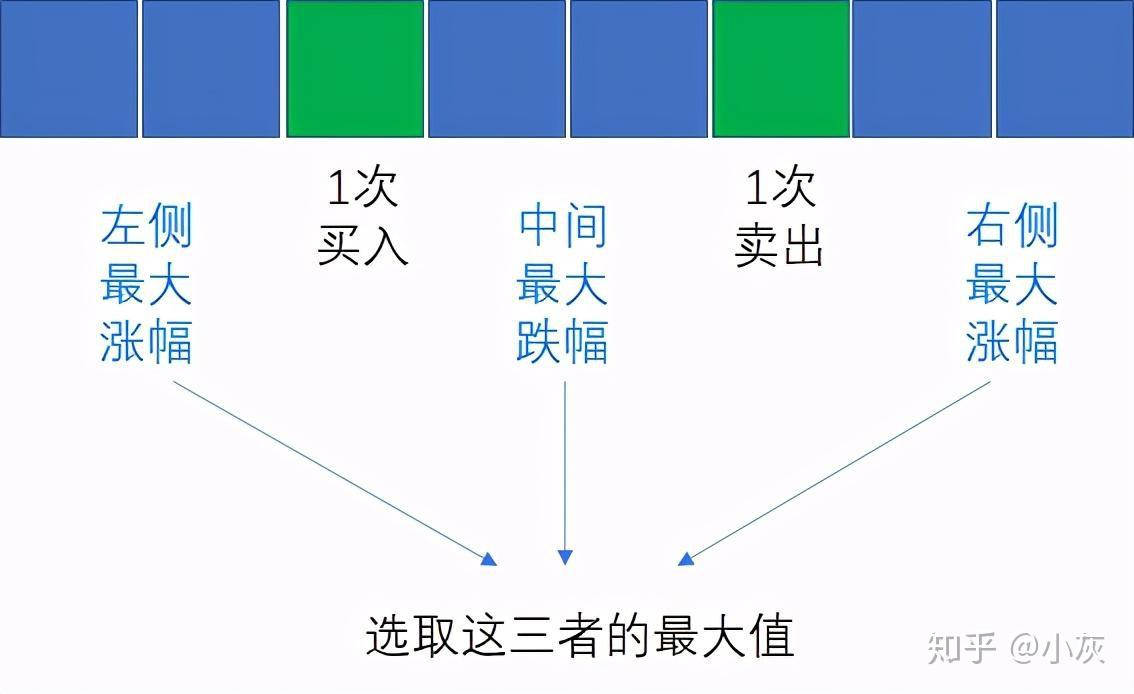It is better to find the largest increase on the left and right Understand, what's the use of looking for the largest drop in the middle?

Think about it and know that when the first trade needs to be When splitting, the maximum decline in the trading range is equivalent to the income increment after splitting (similar to the bottom-hunting operation of stock trading):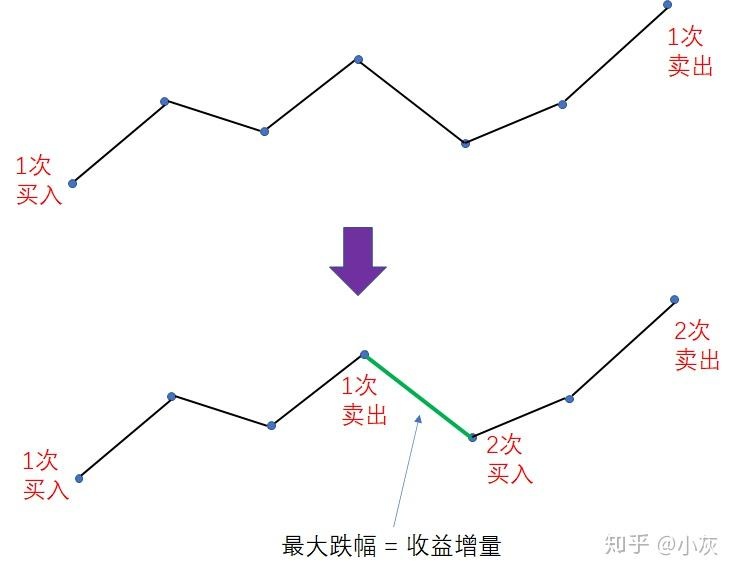In this way, we can summarize the following formula:

Maximum total profit = 1st maximum profit + Max (maximum increase on the left range, the largest decrease in the middle, the largest increase in the right)The so-called dynamic programming is to The complex problem is simplified into smaller sub-problems, and then recursively from the bottom to the top of the simple sub-problem, and finally the optimal solution of the complex problem is obtained.

First of all, let us analyze the current stock trading problem, which requires a certain number of days and certain transactions Maximum profit under the limit of times.

Since the stock trading is limited to a maximum of 2 times, the stock trading can be divided into 5 stages:

First sell

Second sell

We set the trading stage of the stock to the variable m (represented by a number from 0 to 4) and the days range to variable n. and weThe maximum profit of the solution, affected by these two variables, is expressed as a function as follows:

Maximum profit = F(n, m) (n>=1, 0

1. If there is no buying and selling, that is, when m=0, the maximum profit is obviously 0, that is,

F(1,0) = 0

2. If there is 1 buy, that is m When =1, it is equivalent to subtracting the stock price of the first day out of thin air, and the maximum return is the negative stock price of the day, that is,

F(1,1) = -price[ 0 ]

3. If there is one buyout, that is, when m=2, the buys and sells are offset (of course, this has no practical significance), and the maximum profit is 0 , that is,

F(1,2) = 0

4. If there are 2 buys , that is, when m=3, the same as m=1,

F(1,3) = -price[ 0 ]

5. If there are 2 sells, that is, when m=4, the same as m=2,

F(1,4)=0

The initial state is determined, let's take a look at the state transition. If the limit of the number of days is increased from n-1 days to n days, So how will the maximum benefit change?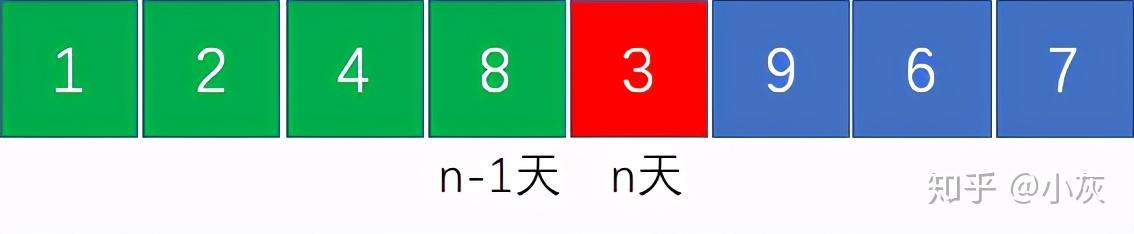It depends on what stage it is now (the number of times to buy and sell), and the operation of the stock price on the nth day (buy, sell or wait and see). Let us analyze the situation at each stage:

1. If there is no transaction before, and the nth day is still waiting, then the maximum profit is still 0, that is, F(n , 0) = 0

2. If there is no previous transaction, and the nth day is bought, then the maximum profit is Negative current stock price, i.e. F(n, 1) = -price[n-1]

3. If there was one purchase before, and the nth day chooses to wait and see, then the maximum profit is the same as before, that is, F(n, 1) = F(n-1, 1)

4. If there was one purchase before and the sale was made on the nth day, then the maximum return is the negative return of the first purchase plus The stock price of the day, that is, then F(n, 2) = F(n-1, 1) + price[n-1]

5. If there was 1 sell before, and the nth day chooses to wait and see, then the maximum profit is the same as before, that is, F(n, 2) = F(n-1, 2)

6. If there was 1 sale before and 2 purchases were made on the nth day, then the maximum profit is the profit of the first sale minus the profit of the first sale The stock price of the day, that is, F(n, 3) = F(n-1, 2) - price[ n-1 ]

7. If there are 2 buys before, and the nth day chooses to wait and see, then the maximum profit is the same as before, that is, F(n, 3) = F(n-1, 3)

8. If there were 2 purchases before and the nth day was sold, then the maximum profit is the profit of the 2nd purchase minus the stock price of the day, i.e. F(n, 4) = F(n-1, 3) + price[n-1 ]

p>

9. If there are 2 sells before, and the nth day chooses to wait and see (and can only wait and see), then the maximum profit is the same as before, that is, F(n, 4) = F(n-1, 4)

Finally, we put the cases [2, 3], [4, 5], [6, 7], [8, 9] combined, can be summarized into the following 5 equations:

F (n, 0) = 0

F(n,1)=max(-price[n-1],F(n-1,1))

p>

F(n,2)=max(F(n-1,1)+price[n-1],F(n-1,2))

F(n, 3) = max(F(n-1, 2) - price[n-1], F(n-1, 3))

F(n,4)=max(F(n-1,3)+price[n-1],F(n-1,4))

From the following 4 equations, the relationship between the maximum benefit of each stage and the previous stage can be summarized:

F(n,m)=max(F(n-1,m-1)+price[n-1],F(n-1,m))

From this we can conclude that the complete state transition equation is as follows: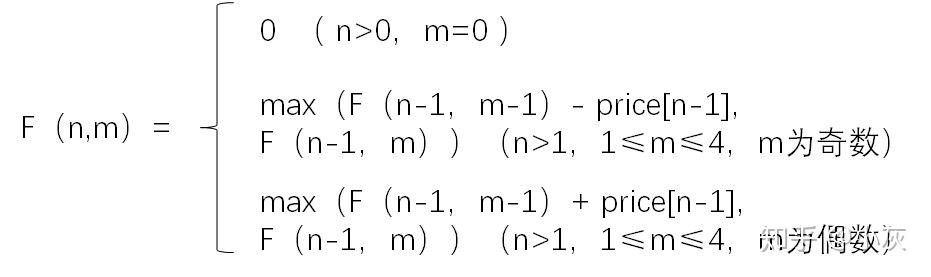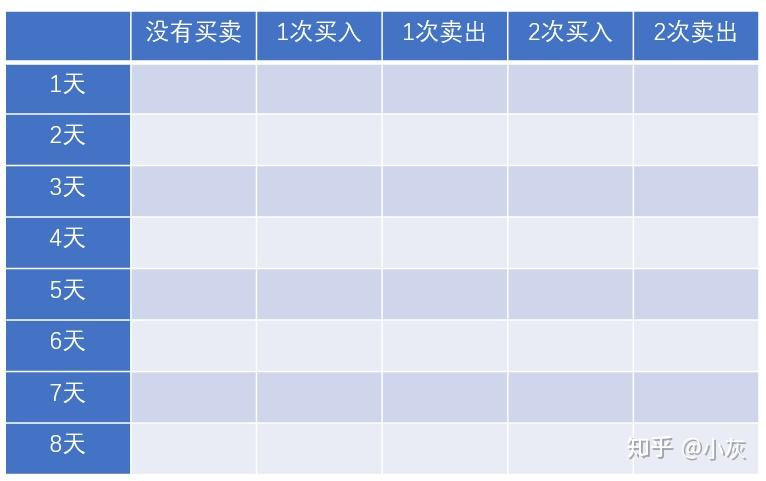In the table, different rows represent the maximum returns under different day limits, and different columns represent the maximum returns at different trading stages.

We still use the array from the previous example to populate the table:First, we populate the table with the initial state: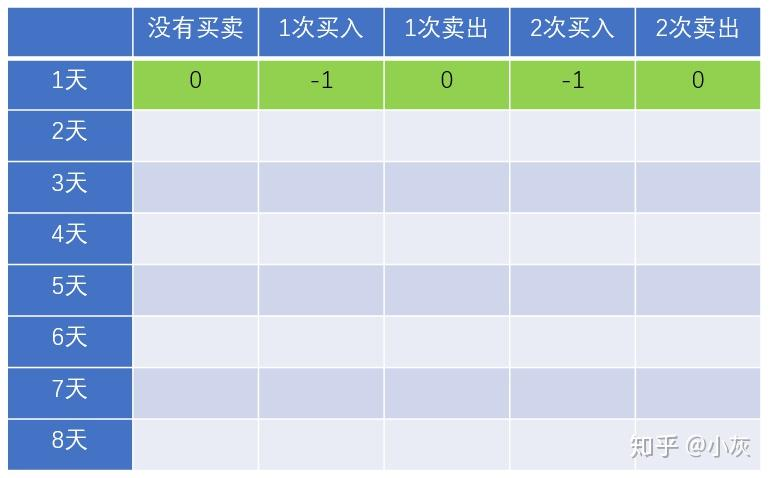Next, we start populating the second row data.

When there is no trading, the maximum profit must be 0, so the result of F(2,0) is 0: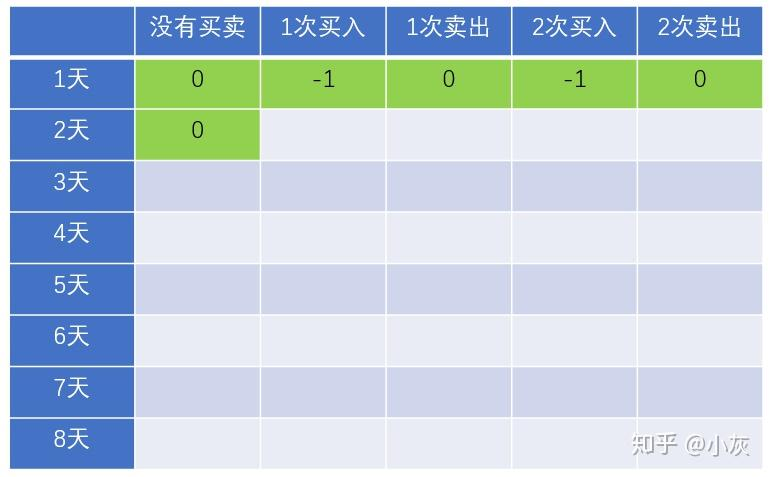According to the previous state transition equation , F(2,1) = max(F(1,0)-2, F(1,1)) = max(-2,-1) = -1, so the result for row 2, column 2 is - 1: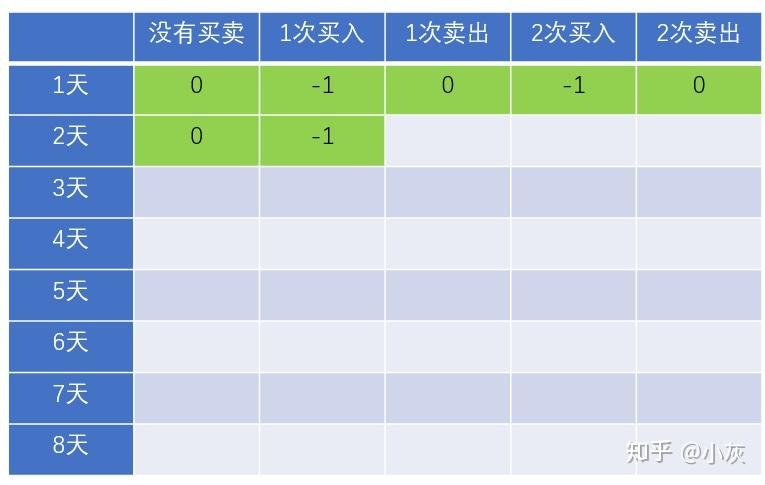F(2 ,2) = max(F(1,1)+2, F(1,2)) = max(1,0) = 1, so the result of row 2, column 3 is 1: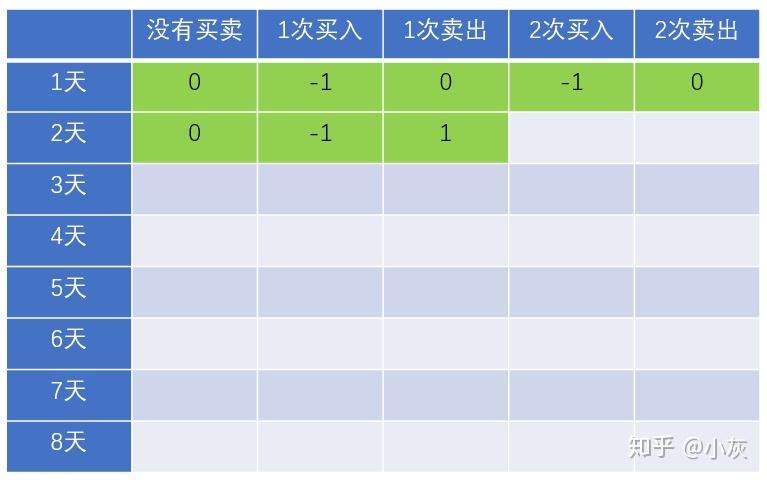F(2,3) = max(F(1,2)-2, F(1,3)) = max(-2,-1) = -1, so the result for row 2, column 4 is - 1: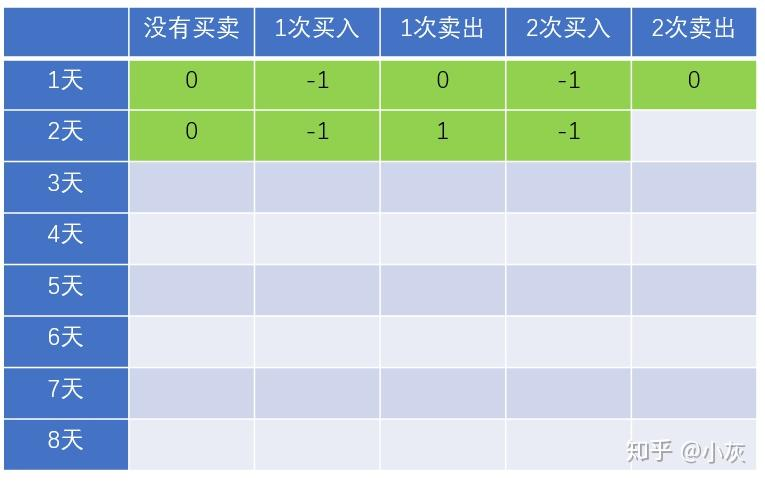F(2 ,4) = max(F(1,3)+2, F(1,4)) = max(1,0) = 1, so the result in row 2, column 5 is 1: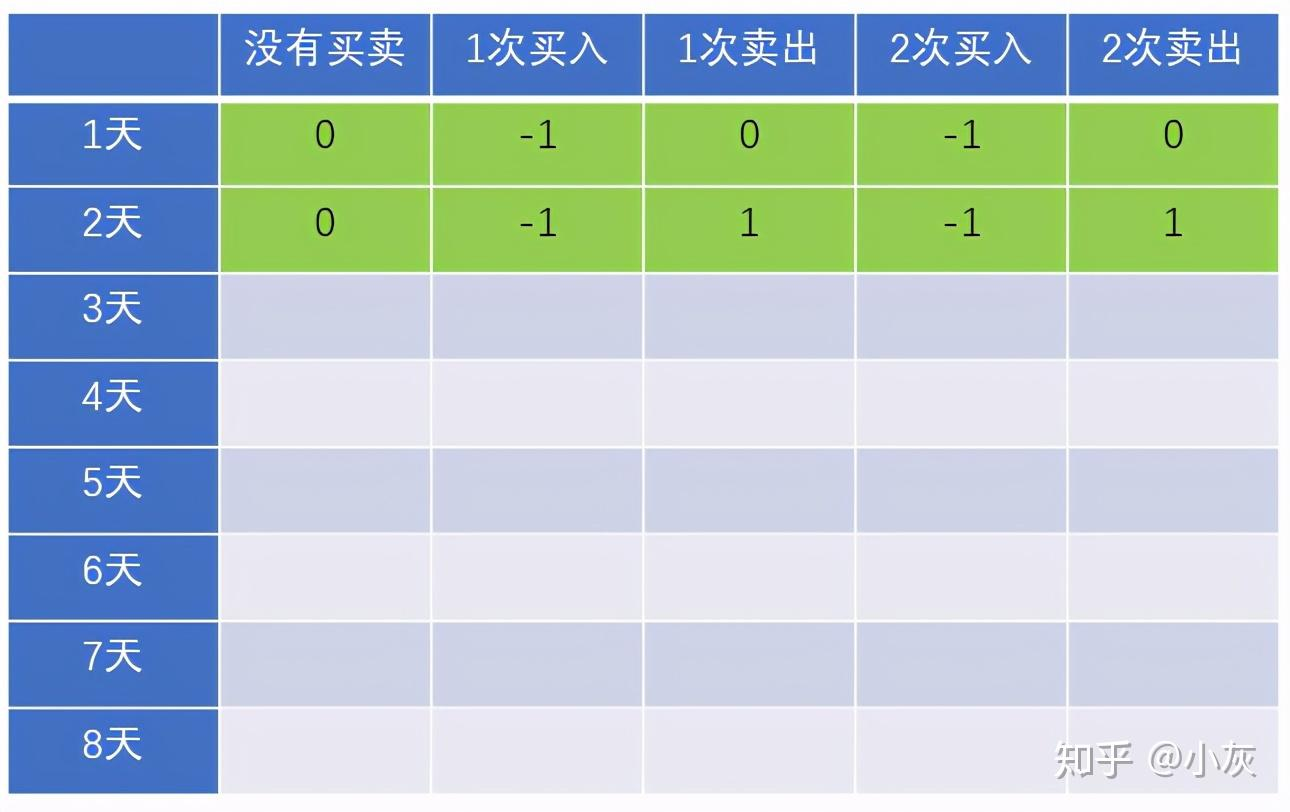Next, we continue to fill in the first 3 lines of data: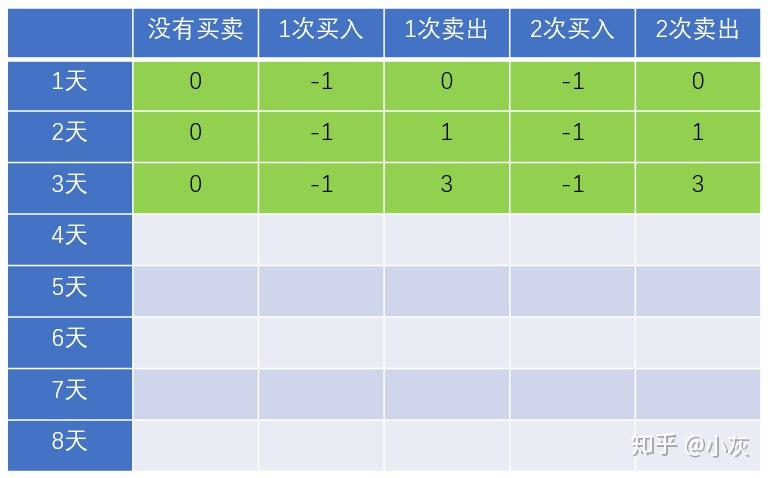Next fill in line 4: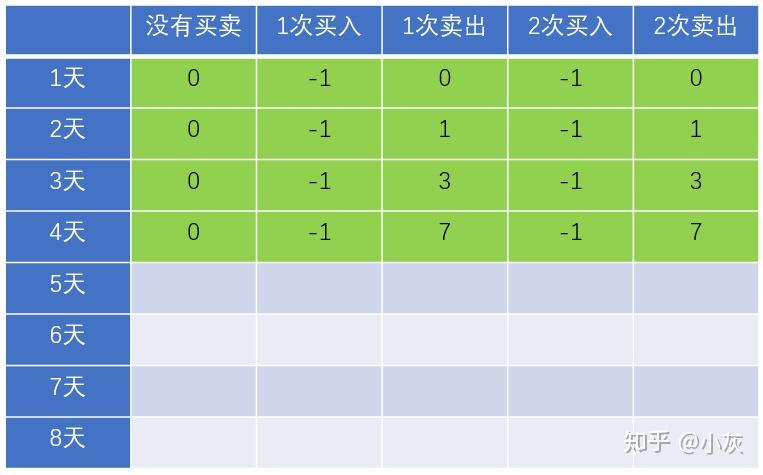And so on, we keep filling the entire table: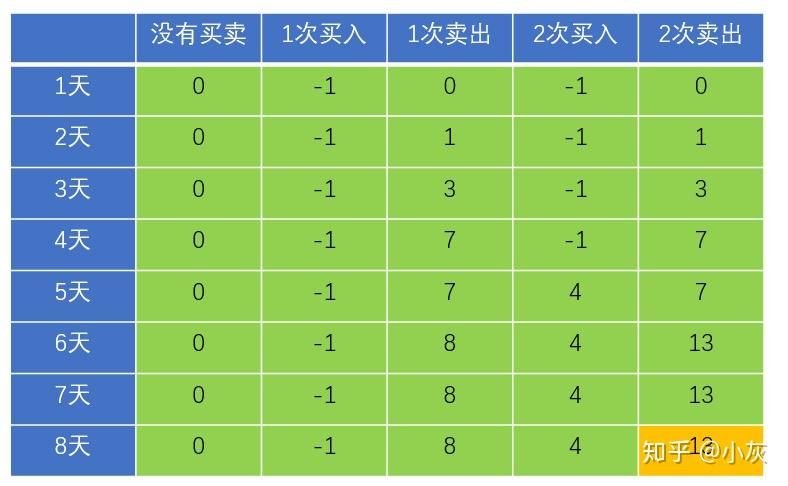As shown in the figure, the last data 13 in the table is the global maximum profit.//Maximum number of trades private static int MAX _ DEAL _ TIMES = 2; public static int maxProfitFor2Time(int[ ] prices) { if(prices==null | | prices.length==0) { return 0; } //Maximum number of rows in the table int n = prices.length; //Maximum number of columns in the table int m = MAX _ DEAL _ TIMES*2+1; //Use Two-dimensional array record data int[ ][ ] resultTable = new int[ n ][ m ]; //fill initial state resultTable[ 0 ][ 1 ] = -prices[ 0 ]; resultTable[ 0 ][ 3 ] = -prices [ 0 ]; //From bottom to top, fill data for(int i=1;i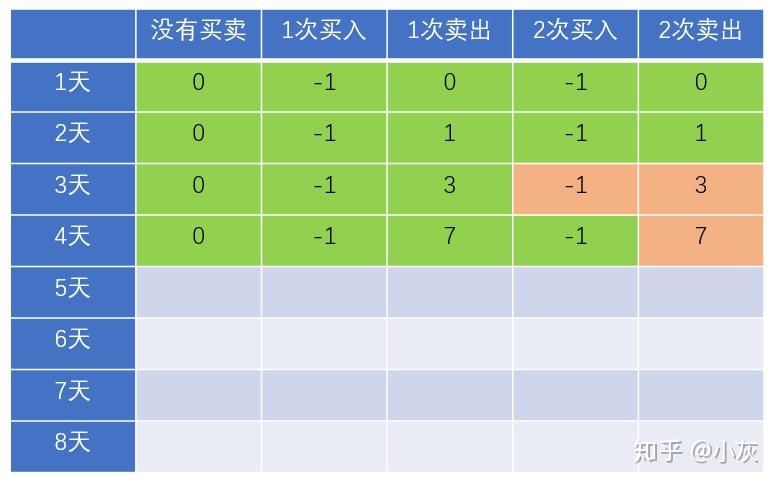//Maximum number of trades private static int MAX _ DEAL _ TIMES = 2; public static int maxProfitFor2TimeV2(int [ ] prices) { if(prices==null || prices.length==0) { return 0; } //Maximum number of rows in the table int n = prices.length; //Maximum number of columns in the table int m = MAX _ DEAL _ TIMES*2+1; //Use a one-dimensional array to record data int[ ] resultTable = new int[ m ]; //fillFill the initial state resultTable[ 1 ] = -prices[ 0 ]; resultTable[ 3 ] = -prices[ 0 ]; //From bottom to top, fill data for(int i=1;ipublic static int maxProfitForKTime(int[ ] prices, int k) { if(prices==null || prices.length==0 || k

Article Url:https://www.liaochihuo.com/info/673221.html

Label group:[stock] [Array formula

• ### CV Morning Read: Jingdong acquires Wuxing Electric; Volvo and Geely merger is suspended; LV launches Apple earphone accessories

Posted on 2020722 100000|Show all floors|Reading mode 1. Jingdong Group acquired the remaining 54% equity of Jiangsu Wuxing Electric Co., Ltd.Confirm...

2022-06-16 Read full >>
• ### The latest version of China's stock market in 2022

Published on May 26, 2022 Author Shanghai Investment Morgan Domestic Demand Power Hybrid Analysis and Discussion of Shanghai Investment Morgan Domest...

2022-06-16 Read full >>
• ### Circulation and production capacity of China's national and urban automakers

Posted on May 26, 2022 Author Chinese Tianying Chinese Tianying00005 full word The distribution and production capacity of OEMs in various province...

2022-06-16 Read full >>
• ### Hong Kong stocks before the market: Technology stocks may be boosted by the outside world, Nai Xue's tea will open the dark market today

Original title Hong Kong stocks before the market Technology stocks may be boosted by the outside world, Nai Xues tea will open the dark market today...

2022-06-16 Read full >>
• ### Who can become a demon in Datang Telecom, Fuchun Shares, and Xinli Finance?

1. Several important votes today Datang Telecom, Fuchun Shares, Xinli Finance These three stocks create an ultrashort atmosphere. Everyone knows t...

2022-06-16 Read full >>
• ### After-hours rise and fall list! The decline of the stocks on the board is too fast, or will there be a strong rebound?

Todays market trend The market opened lower today, and Fridays market was relatively poor during this time a day. Previously, the market was oscillat...

2022-06-16 Read full >>
• ### Geely Equity Financing, Development Equity Financing

To talk about the skills of stock trading, I think the biggest skill is how to read the market, because reading the market is equivalent to knowing a...

2022-06-16 Read full >>
• ### The "new crown market" is far from over, these 9 stocks are expected to continue to benefit?

As we finally close this 2020 book, we will never forget what happened when the coronavirus pandemic turned our world upside down. In the meantime, we...

2022-06-16 Read full >>
• ### A shares suddenly dived in late trading, what happened? The brokerage leader has 6 days and 5 boards, and this indicator is triggered!

Original title A shares suddenly dived in late trading, what happened? The brokerage leader has 6 days and 5 boards, and this indicator is triggered ...

2022-06-16 Read full >>
• ### What are the leading photovoltaic stocks, a list of leading photovoltaic stocks

Photovoltaic power generation mainly refers to a device that relies on solar energy to generate electricity. Its power generation principle is to us...

2022-06-16 Read full >>